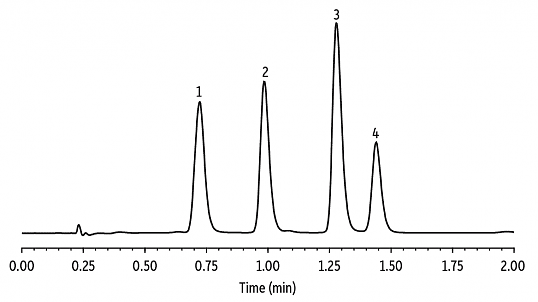# Chromatographic Resolution

1. The following data were obtained for four compounds separated on a 20-m capillary column.

compound

tr (min)

w (min)

A

8.04

0.15

B

8.26

0.15

C

8.43

0.16

Calculate the resolution and relative retention values for each pair of adjacent compounds. The retention time for an unretained solute is 1.19 min.

1. Using the chromatogram shown below, calculate the retention factor for each peak 1-4 and find the resolution and relative retention for each pair of adjacent compounds. (Hint: use a ruler!)1. Using the partial chromatogram below, determine the resolution between the two solute bands. (Hint: you’ll need a ruler!)1. Suppose you want to increase the resolution between the two components to 1.5.
1. What difference in retention times is necessary to achieve this resolution assuming the peak widths do not change?

2. If the difference between the retention times remains the same, how would the peak widths have to change?

1. The following data were obtained for four compounds separated on a 20-m capillary column.

compound

tr (min)

w (min)

A

8.04

0.15

B

8.26

0.15

C

8.43

0.16

1. Calculate the number of theoretical plates for each compound and the average number of theoretical plates for the column.

2. Calculate the average height of a theoretical plate.

1. The partial chromatogram below was obtained on a 2-m column with tm= 50 s. Suppose you want to increase the resolution between the two components to 1.5. Without changing the height of a theoretical plate, what length column do you need? What height of a theoretical plate do you need to achieve a resolution of 1.5 without increasing the column’s length?1. The van Deemter plots shown below correspond to packed columns that are identical except for the size of the stationary phase particles (3, 5, and 10 μm).1. Which column would show the best resolution for two similar solutes? What aspect(s) of the graph above allowed you to make this decision?

2. Use the three terms in the van Deemter equation to explain the differences in these plots.